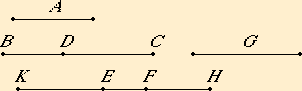# Proposition 112

The square on a rational straight line applied to the binomial straight line produces as breadth an apotome the terms of which are commensurable with the terms of the binomial straight line and moreover in the same ratio; and further the apotome so arising has the same order as the binomial straight line.

Let A be a rational straight line, let BC be a binomial, let DC be its greater term, and let the rectangle BC by EF equal the square on A.

I say that EF is an apotome the terms of which are commensurable with CD and DB, and in the same ratio, and further, EF has the same order as BC.

Again let the rectangle BD by G equal the square on A.Since, then, the rectangle BC by EF equals the rectangle BD by G, therefore CB is to BD as G is to EF. But CB is greater than BD, therefore G is also greater than EF.

V.17

Let EH equal G. Then CB is to BD as HE is to EF, therefore, taken separately, CD is to BD as HF is to FE.

V.12

Let it be contrived that HF is to FE as FK is to KE. Then the whole HK is to the whole KF as FK is to KE, for one of the antecedents is to one of the consequents as the sum of the antecedents is to the sum of the consequents.

V.11

But FK is to KE as CD is to DB, therefore HK is to KF as CD is to DB.

But the square on CD is commensurable with the square on DB, therefore the square on HK is commensurable with the square on KF.

And the square on HK is to the square on KF as HK is to KE, since the three straight lines HK, KF, and KE are proportional. Therefore HK is commensurable in length with KE, so that HE is also commensurable in length with EK.

Now, since the square on A equals the rectangle EH by BD, while the square on A is rational, therefore the rectangle EH by BD is also rational.

X.20

And it is applied to the rational straight line BD, therefore EH is rational and commensurable in length with BD, so that EK, being commensurable with it, is also rational and commensurable in length with BD.

X.11

Since, then CD is to DB as FK is to KE, while CD and DB are straight lines commensurable in square only, therefore FK and KE are also commensurable in square only. But KE is rational, therefore FK is also rational.

X.73

Therefore FK and KE are rational straight lines commensurable in square only, therefore EF is an apotome.

Now the square on CD is greater than the square on DB either by the square on a straight line commensurable with CD or by the square on a straight line incommensurable with it.

X.14

If the square on CD is greater than the square on DB by the square on a straight line commensurable with CD, then the square on FK is also greater than the square on KE by the square on a straight line commensurable with FK.

And, if CD is commensurable in length with the rational straight line set out, then FK is also; if BD is so commensurable, then KE is also; but, if neither of the straight lines CD nor DB is so commensurable, then neither of the straight lines FK nor KE is so.

X.14

But, if the square on CD is greater than the square on DB by the square on a straight line incommensurable with CD, then the square on FK is also greater than the square on KE by the square on a straight line incommensurable with FK.

And, if CD is commensurable with the rational straight line set out, then FK is also; if BD is so commensurable, then KE is also; but, if neither of the straight lines CD nor DB is so commensurable, then neither of the straight lines FK nor KE is so, so that FE is an apotome, the terms of which, FK and KE are commensurable with the terms CD and DB of the binomial straight line and in the same ratio, and it has the same order as BC.

Therefore, the square on a rational straight line applied to the binomial straight line produces as breadth an apotome the terms of which are commensurable with the terms of the binomial straight line and moreover in the same ratio; and further the apotome so arising has the same order as the binomial straight line.

Q.E.D.

## Guide

This proposition is not used in the rest of the Elements.